# S7E1 - Electromagnetic Waves, Frequency Calculations, and Wavelength

Before we can address the nature of the atom, we must first discuss some similar properties of light...

Light - also called "Electromagnetic Radiation."

==========

Energy, in the form of "light," travels through space by electromagnetic radiation.

There are only seven types of EMR which together make up the electromagnetic spectrum.

==========

## The Electromagnetic Spectrum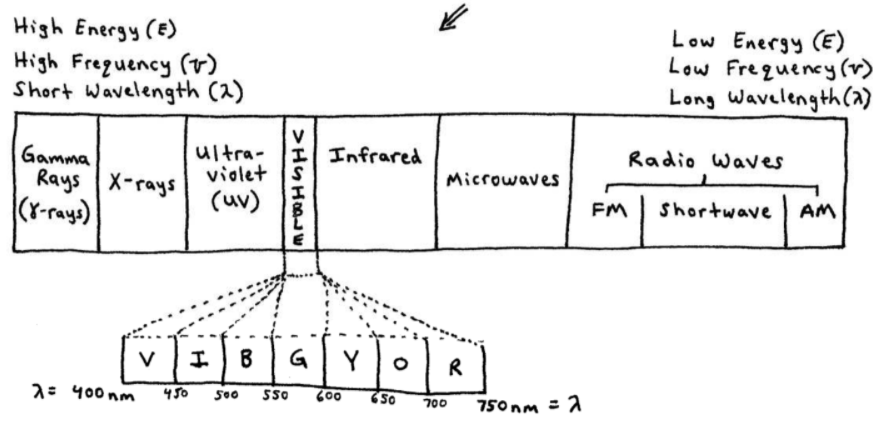==========

## Electromagnetic Spectrum - Notes

1.  Light (EMR) only differs in its energy, frequency, and wavelength.

2.  Visible light only makes up a "sliver" of the electromagnetic spectrum.

3.  Soon we will see the mathematical relationship between energy (E), wavelength (λ), and frequency (ν).

4.  To remember the complimentary colors for visible light, use (and sound out):## " VY BO GR "

➞ If violet light is absorbed, objects appear yellow.

➞ if yellow light is absorbed, objects appear violet.

➞ etc.. for the other pairs...

➞ we'll drop / ignore "I" (indigo)

==========

## Wavelength and Frequency of Light

Check out the two waves in the drawing below.  But first, a few definitions:

Wavelength (λ) - distance between two consecutive peaks (or troughs) in a wave.

Frequency (ν) - the number of cycles (waves) per second traveling past a fixed point.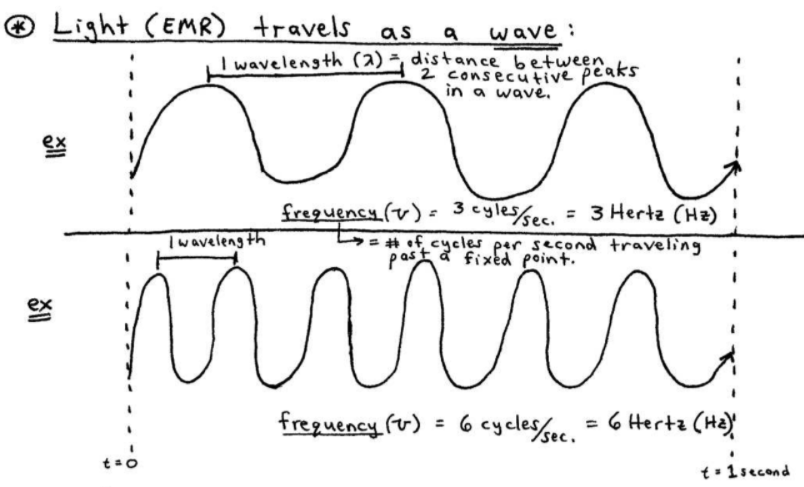As you can see above, the longer the wavelength, the lower the frequency, and vice-versa.

To put this visually we have the mathematical relationship between frequency and wavelength...

==========

## Wavelength and Frequency RelationshipIn fact, multiplying the wavelength (λ) times the frequency (ν) will always equal the same value.

And this value is the speed of light (c)

c = 2.9979 x 108 meters/second.

Often you'll see c = 3.0 x 108 m/s...

----------

## Frequency Wavelength Formula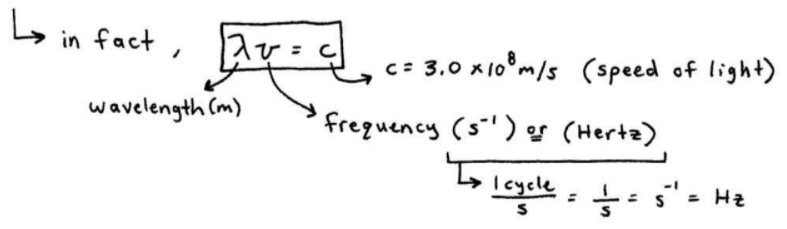Let's make sense of this by doing a sample problem using  λν = c  below...

----------

## Frequency Wavelength Calculation

ex:  Calculate the frequency of red light having a wavelength of 6.50 x 102 nm.
_________

➞ first, we'll need to convert our wavelength from nanometers to meters.

➞ so, "start with what we're given and put it over 1"...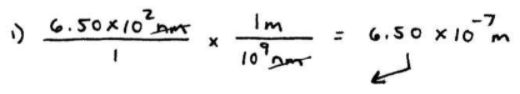Okay good.  Now we can actually DO the problem: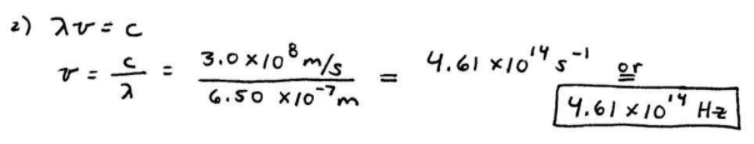==========

Next up, in SECTION 7 - Quantum-Mechanical View of the Atom, and Periodicity...

We'll see a video and discuss the wave-particle duality of light, the de Broglie wavelength, and the photoelectric effect...  👍